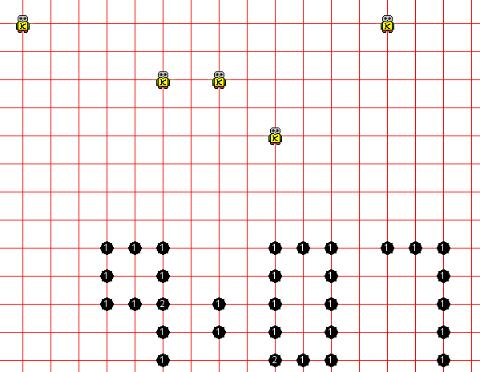### Digital Clock 2

6 points

This program is an improvement of your digital clock program. Here, the program reads the time from the computer clock and displays that time. The code to read the time from the computer clock is provided to you, in main().

Create 5 robots in main(), just as you did in the first program.

You will need to declare 4 integers to store the time's digits (for many times only 1 digit is needed to display the hour). The hour is stored in the integer hour and the minute is stored in the integer minute. Write code to figure out what 4 digits you want to display. Remember that modular arithmetic with 2 integers gives you the remainder; for example 7 % 3 equals 1. Recall also that integer division with 2 integers gives you the quotient without the remainder; for example 7 / 3 equals 2.

For each robot, make a call to drawDigit(), sending in to drawDigit() the correct digit to draw.

Write the method drawDigit() to draw 1 digit. You will want to use an IF - ELSE IF structure in drawDigit() to make the correct method call, based on the number sent into drawDigit().

Here is an image that shows the time 9:07 being displayed by my digital clock program: